Congruence of Triangles

SAS, SSS, ASA, AAS and RHS Theorem

Congruence is a term used to define two geometrical figures on a plane that are the exact same. It can be the mirror image of the given geometric figure or the rotation of the given shape. They can superimpose on each other, as the line segments that they are drawn with are of the same length and their internal angles are the exact same. Two sets of points can be called congruent if they can be transformed into the other by collectively moving them with a set of rigid motions.

Congruence of Triangles:

A triangle is a polygon formed by three-line segments joining and forming three internal angles. Two triangles can be said to be congruent if their corresponding sides are equal in their length and their corresponding angles are equal in their measure. For example, if there are two triangle, Δ ABC and Δ DEF, where AC = DF, BC = EF, AB = DE, and A =D, B = E, C = F, we can say Δ ABC is congruent to, ΔDEF.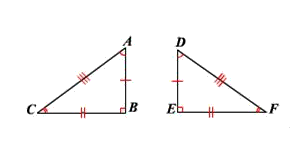This is written as Δ ABC , ΔDEF,

Two congruent triangles can be made to look different from each other through

Rotation: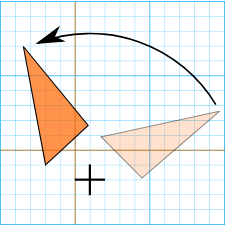Reflection: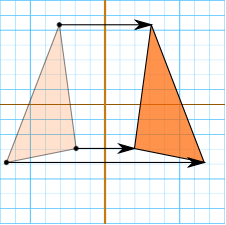Translation: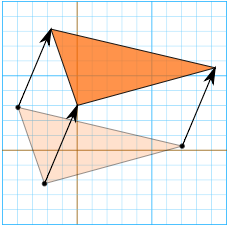Proving Triangles are Congruent:

A triangle can be identified by its six measures, which are three angles and three sides. Two triangles are congruent if all these six measures are equal. But while trying to determine congruence, if we have three particular specific measures it will be enough.

There are five theorems which can be applied while trying to determine congruency of triangles

• 1) SSS theorem (Side side-side theorem)

• 2) SAS theorem (Side angle side theorem)

• 3) ASA theorem (Angle side angle theorem)

• 4) AAS theorem (Angle angle side theorem)

• 5) RHS or HL theorem (Right angle Hypotenuse Side or Hypotenuse Leg theorem)

• Here is how the theorems work

• 1) SSS theorem (Side side-side theorem)

• The SSS theorem states that if three sides of a triangle are equal in length to three corresponding sides of other triangles, then the two triangles are congruent.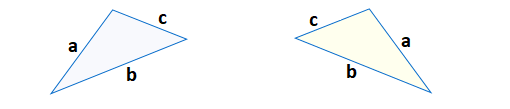In the above two triangles, the sides marked a, b, and c are the same in length. We can, therefore, conclude that the above two triangles are congruent.

• 2) SAS theorem (Side angle side theorem)

• The SAS theorem states that if in any two triangles, two sides and the angle between them in one triangle is the same as two sides and the angle between them in the other triangle, then the two triangles are congruent.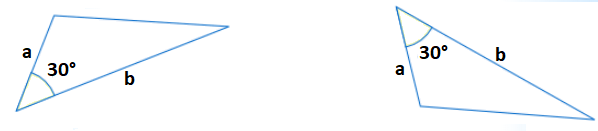In the above two triangles, the sides marked a and b in both triangles are equal to each other, and the angle between them is 30° in each case. Hence the triangles are congruent.

• 3) ASA theorem (Angle side angle theorem)

• The ASA theorem states that if in any two triangles, two angles and the side between the two angles in one triangle is equal to two angles and the side between those two angles in the other triangle, then the two triangles are congruent.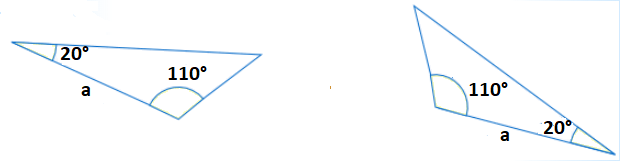In the above two triangles, there are angles which measure 20 ° and 110 ° with a side marked a which is the same in length in both triangles. Hence the triangles are congruent.

• 4) AAS theorem (Angle angle side theorem)

• The AAS theorem states that if in any two triangles, two angles and any side which is not between them in one triangle is equal to two angles and the corresponding side in another triangle, the two triangles are congruent.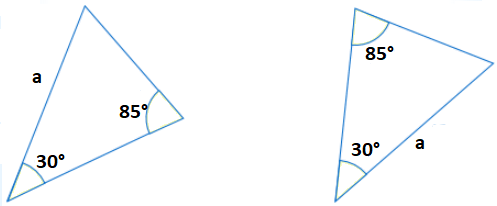In the above two triangles, the two angles are 20 ° and 110 ° respectively and one of the non-included sides, marked a is the same in both triangles. Hence the triangles are congruent.

• 5) RHS or HL theorem (Right angle Hypotenuse Side or Hypotenuse Leg theorem)

• This theorem applies only to right-angled triangles. If in any two triangles, the hypotenuse and a side of one triangle are equal to the hypotenuse and side of another triangle, the two triangles are congruent.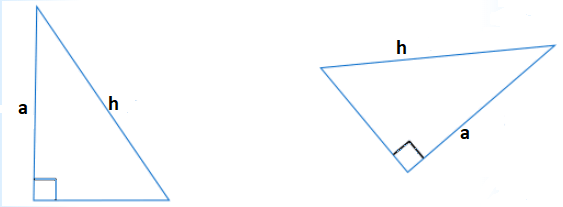In the above two triangles, the hypotenuse of both the triangles, marked h and a side of the triangle marked a, are equal. Hence the triangles are congruent.

It is not possible for any three set of data to determine if the given triangles are congruent. For example, if two sides an angle which is not between them is the same as two sides and the corresponding angle in another triangle, it is not necessary that the triangles are congruent. Here are some commonly confusable set of data and why they cannot be used to determine congruency.

• 1) AAA theorem (Angle angle-angle theorem)

• If all the angles of any two triangles are the same, the triangles will possess the same shape, but it is not necessary that they are congruent. For example,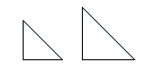The above two triangles have the same angles and general shape, but they are not congruent as the length of their sides is different.

• 2) SSA theorem (Side side angle theorem)

• If two sides and an angle which is not between those two sides are equal in any two triangles, the two triangles are not necessarily congruent. For example,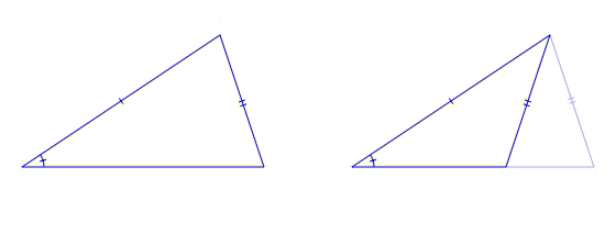The above two triangles have one angle which is the same, and two sides which are the same. But we can see from the second triangle that a non-congruent triangle with the same angle and sides may exist.

Properties of Congruent Triangles:

If we can establish that two given triangles are congruent, then we can say that all corresponding sides and angles of the two triangles are the same. Hence if we prove that two triangles are congruent using any one of the congruency theorems, we can find the measurement of angles or sides of one triangle if we know the corresponding measurements in the other triangle which is congruent to it.

For this we use the acronym CPCT, which stands for Corresponding Parts of Congruent Triangles, So if we can establish that two triangles are congruent and we know measurements of one triangle, by CPCT we can assume the measurements of the other triangles.

Also, if two triangles are congruent, other than the angles and sides, all other properties of the triangles, like the perimeter, center of the triangle, etc are all the same.

Problems related to Congruency of Triangles:

• 1) In the figure given below, ABCD is a parallelogram. BEFC is a square. Prove that the two triangles ABE and DCF are congruent to each other. Also, prove that AE = DF.

•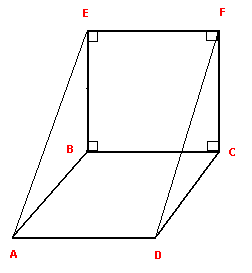Solution: In the given figure, BA = CD, as they are opposite sides of a parallelogram.

EB = FC as they are opposite sides of a square.

Also, since the side, EB is parallel to FC (opposite sides of a square) and BA is parallel to CD (opposite sides of a parallelogram) then EBA = FCD. Since EBA is between EB and AB while FCD is between FC and DC, we can conclude by SAS theorem that Δ ABE Δ DCF.
Hence by CPCT, we can conclude that AB = DF.